# The Missing Knob on Your Random Vibration Controller

#### Author Philip Van Baren

Random vibration testing is the industry workhorse for simulating the environment for a broad range of products. Tests are typically specified by defining a spectrum shape and overall RMS amplitude. The test controller then causes a measured reference acceleration to match these specified parameters. The controller forces a shaped-random response with a normal or Gaussian amplitude distribution. However, experience has shown that such tests may be too conservative for some product/environment combinations. The test does not produce the same damage statistics observed in the field. Adding a third control dimension provides more realistic random vibration tests that better match the damage potential of the actual environment. That third dimension is kurtosis control, which matches the amplitude distribution of the test to that of the environment.

Random vibration testing as used today is largely unchanged in technique since it was introduced in the early 1950s. It attempts to capture the essence of the service vibration environment for a product and reproduce a similar environment in your test lab. It does this by summarizing the test environment using a frequency spectrum, which gives the relative weighting of each frequency band and an averaged overall signal intensity. The frequency spectrum is typically in terms of acceleration power spectral density (PSD) and the overall signal intensity is defined as the root-mean-squared (RMS) averaged acceleration.

The primary advantage of random vibration testing is that it produces a waveform similar in appearance to those actually measured in the field. Despite the similarity of random test and environmental waveforms, it is increasingly recognized that current random test specifications do not capture the field vibration environment with sufficient intensity for many tests. What is the problem with current random techniques? Our experience suggests very strongly that the problem is the inability to reproduce peak accelerations which occur in actual use of a product.

One proposed method to rectify this situation is to use a field data replication (FDR) technique, where the actual waveform measured in the field is reproduced on a shaker in the lab. This method can be extremely useful for many tests. But critics claim that, since the waveform produced in the test is always the same as the field measurement, it doesn’t capture the variability that can occur in the field. For example, each lap around the track will produce a different vibration waveform, so simply recording a single lap and repeating that lap many times on your shaker removes the variability. Also, the large amount of data involved makes it difficult to define a standard, and makes it difficult to define pass/fail criteria for the test. Because of this, there are still very few test specifications based on FDR techniques.

You may have experienced the inability of random vibration tests to produce peak accelerations seen in the real world. In fact, you may have wished that you could run a shock-on-random test, because you have experienced these very limitations. If this has piqued your interest, read on to learn more about a term you have not heard about since your last statistics class – kurtosis.

### What Is Kurtosis?

Let’s take a look at what kurtosis is, and what is important to know about this variable when running a random vibration test. In what follows, we will use kurtosis in its normalized The Missing Knob on Your Random Vibration Controller form. Normalized kurtosis is defined as the fourth statistical moment divided by the square of the second statistical moment. This is done to remove variability due to waveform amplitude from the measurement. In this normalized form, the kurtosis for Gaussian data is always 3, regardless of the RMS level or PSD. The normalized kurtosis value K can be easily computed from N samples of zero-mean waveform data, xi , using Equation 1.Now, let’s consider some typical “real world” data. To do this, we measured acceleration on an automotive dashboard while driving on a gravel road. These measurements were imported into VRC’s RandomVIEW software, which calculated an average PSD and kurtosis of the measured data. From these measurements, the kurtosis was evaluated to be 3.8, indicating this environment was not Gaussian.

Then we used a vibration controller to run two random tests intended to match this environment. Both tests were controlled to the same measured PSD spectrum and overall RMS values. In one test, a standard algorithm producing a Gaussian signal was used. In the second, a kurtosis control algorithm was used to match the measured 3.8 value.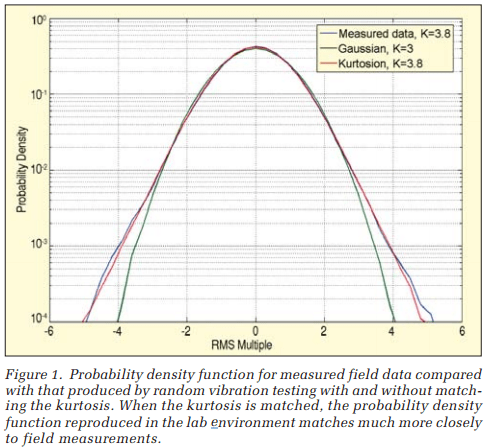As shown in Figure 1, the PDF of the kurtosis-controlled test produced a far better match to the field measurements than the Gaussian-controlled test. Note that the field measurement and kurtosis-controlled test both exhibit broader PDF ‘tails’ than the Gaussian test. These tails indicate the measured environment was more severe than the Gaussian test intended to represent it.

### Real-World Statistics

Before we dive into the details of controlling kurtosis in the test lab, it is useful to examine some real-world data. To this end, we made a series of 40 measurements in four different environments:

• Aeronautical: 2004 Cessna Skylane 182 Turbo
• Agricultural: TS110 New Holland Tractor and Celery Harvester
• Industrial: Ground Heater-Diesel, single-cylinder generator, Kubota engine

Accelerometers were mounted on various locations (windows, dashboards, armrests, steering column, power take off motor, loader frame, engine bracket, etc.), and the vibration environment was measured using our RecorderVIEW software. The kurtosis values computed for all 40 measurements are shown in Figure 2. Of the 40 different data sets, 58% of the data had a kurtosis level greater than 3.3, for which kurtosis reproduction will have a significant effect.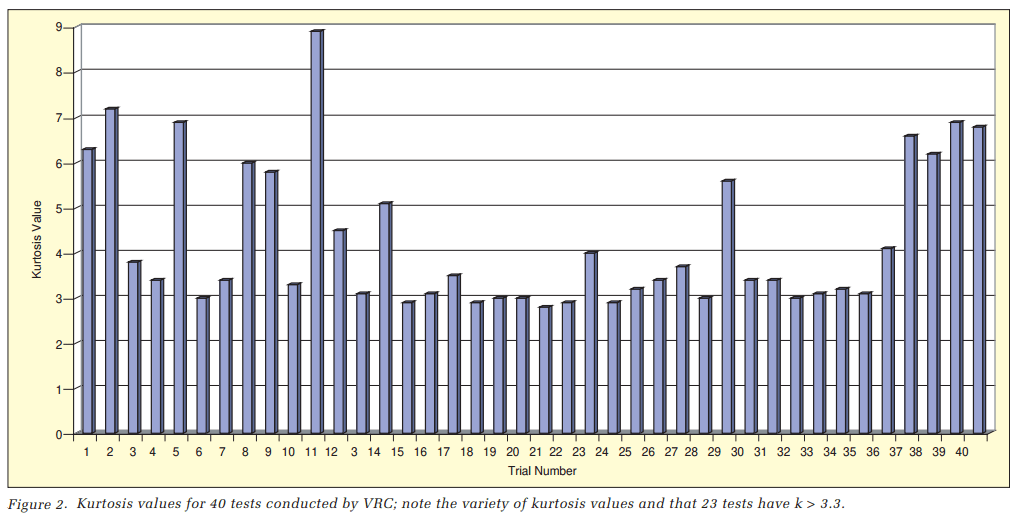Our results corroborate previous results of others.1,2,3 While a Gaussian probability density function may be sufficient in some cases, the field data are often significantly non-Gaussian. The difference is in the the amount of time spent at or near the peak levels. The Gaussian distribution is concentrated around the central, or mean, value (typically around zero), with relatively little time spent at or near peak levels. In fact, a Gaussian waveform will instantaneously exceed three times the RMS level only 0.27% of the time.

When measuring field data, the situation can be considerably different, with amplitudes exceeding three times the RMS level as much as 1.5% of the time. This difference can be significant, since it has also been reported1 that most fatigue damage is generated by accelerations in the range of two to four times the RMS level. Significantly reducing the amount of time spent near these peak values by using a Gaussian distribution can therefore result in significantly reducing the amount of fatigue damage caused by the test relative to what the product will experience in the real world.

### Industry History

The Gaussian distribution has been used in random testing since this method was first established in the 1950s. There are several reasons for this. First, linear filtering of one Gaussian distribution will result in another Gaussian distribution. So spectrum shaping and the shaker frequency response function do not change the amplitude distribution. This linear transformation property also means that Gaussian data in the time domain transformed through an FFT (as is done in digital random control systems) will result in Gaussian distributions in the frequency domain.

Secondly, a Gaussian distribution can be completely determined by two parameters – the mean and standard deviation.

In a random vibration context the mean (the average acceleration) is always zero. Therefore, the Gaussian distribution of a standard random vibration test can be completely defined using a single parameter – the standard deviation (the RMS acceleration).

However, considering data that suggest inadequacies in the Gaussian distribution in representing real-world data, it is useful to consider additional parameters. Ideally a single additional number could be added to the test specification to capture the probability density distribution for the peaks. What mathematical manipulation could possibly do this for us? In terms of statistical parameters, the mean is the first statistical moment, and the RMS is the square root of the second statistical moment. So an obvious extension is to consider matching additional statistical moments between the lab distribution and the distribution of the field data. For symmetric distributions centered around zero, the third statistical moment (also called the ‘skewness’) will be zero. The fourth statistical moment (also called the kurtosis) is sensitive to the increased probability of peaks. In fact, this parameter is also sometimes referred to as the ‘peakyness’ factor, and as such, is a desirable parameter to include in the random controller to better match the PDF of the field data. In fact, it may even be a required parameter for a random test to be realistic.

It has been suggested in the past1 that this kurtosis value can be used to capture the difference between field statistical distribution and the lab distribution. Two different methods of integrating kurtosis into vibration controller systems have been suggested previously.2,3 The former method was not implemented in commercial systems, since it was not technically feasible to implement with current controller technology. The latter, while technically feasible to implement, changes the statistical distribution through the use of a nonlinear element, and this nonlinearity can significantly reduce the dynamic range of the resulting control system. Also, since the nonlinear function operates on a sample-by-sample basis, the resulting change in statistical distribution is not reflected over the full frequency range.

What is desired is an extension of the random test method in which the following properties hold for the resulting acceleration signal:

• The PSD can be controlled as in standard random tests
• The RMS level can be controlled (which automatically follows from proper spectrum control)
• The kurtosis level can be controlled
• The kurtosis level is increased over the full test bandwidth
• The dynamic range of the control system is maintained

### Development

Vibration Research Corporation has developed a new patentpending technique to do random control with precisely these properties. We call this technique ‘kurtosion,’ where, in addition to the standard random test PDF and RMS parameters, a kurtosis parameter is defined to produce a test in the lab. This is similar to current random tests but is one step closer to the vibrations measured in the field. This kurtosis parameter can be easily measured from field data using Equation 1 in the same manner as the RMS and PDF are currently determined from field data.

This technique begins with a statistical distribution that is similar in shape to the distributions seen in field-measured data and which has a variable kurtosis level controlled by a single parameter. The distribution used is plotted in Figure 3 for a sampling of kurtosis values. As can be seen in Figure 3, the ‘tails‘ of the distribution are extended to achieve the increased kurtosis the same way as the ‘tails’ are extended in non-Gaussian field measurements.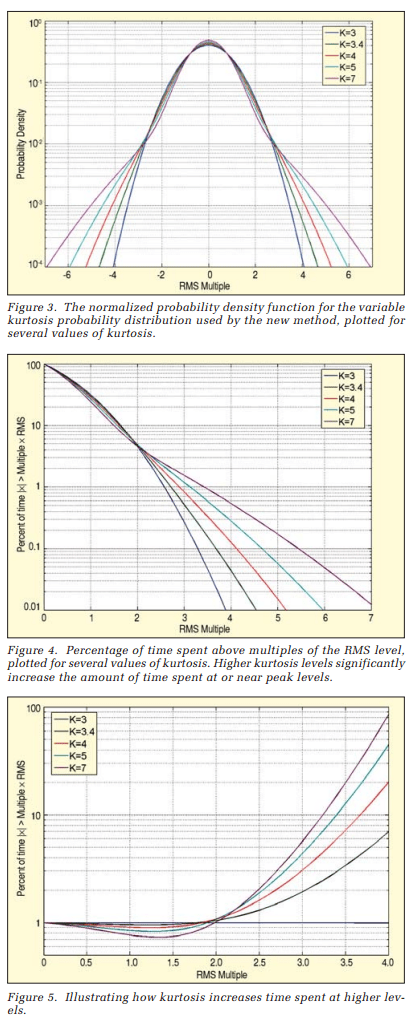The kurtosis parameter shifts the distribution smoothly over the full range from Gaussian through high kurtosis levels. A feedback control loop can be implemented in the controller to achieve the desired kurtosis level in the acceleration waveform. It is important to implement feedback control on the acceleration measurements, because the kurtosis level at the controller output will typically be different from that in the acceleration waveform. Without the feedback control, you could set the kurtosis of the controller output signal, but this would not achieve the desired kurtosis on your shaker.

An alternative view of the distribution is plotted in Figure 4, where the probability of exceeding an absolute-value amplitude is shown. This view is interesting because it is readily evident that the kurtosis parameter directly translates to an increase in the time spent at higher amplitudes. Note that the traces cross at twice the RMS value, with a probability of 4.6%. For the chosen family of distributions, regardless of the kurtosis, the instantaneous acceleration is less than twice the RMS value for 95.4% of the test time. The most damaging events occur at higher levels that represent less than 5% of the test duration. Kurtosis strongly affects the severity of these events.

For example, a Gaussian distribution (kurtosis = 3) spends only 0.27% of the time above three times the RMS level. Increasing the kurtosis to four increases this time to 0.83%. And increasing the kurtosis to seven increases this time to 1.5%. This may not sound like much, but consider that during a 1- hour test, the test article will be approaching a full minute at levels above three sigma, rather than below three sigma. This statistic can translate to significant product stresses. These stresses will not occur with a traditional Gaussian distribution but do occur in a real environment.

To illustrate this point, we divide each vertical amplitude in Figure 4 by the amplitude for K = 3 (the Gaussian case) to get the relative time above absolute value versus RMS multiple for each value of K. This is plotted in Figure 5, where we can clearly see that with increased kurtosis, the relative amount of time spent at the higher levels is increased many times over the Gaussian case.

A second aspect of the technique is that the PSD and RMS levels can be controlled in a manner similar to standard random tests. Any tests previously run with standard random controller systems can now also be run with the same PSD specifications with a user-defined kurtosis value. Not only does the new technique maintain the same frequency spectrum, it also operates so that the kurtosis of the acceleration waveform can be adjusted without affecting or distorting the frequency spectrum. As a result, the kurtosis feedback control loop can operate independently from the standard random spectrum control without any effect on the spectrum.

A third aspect of the technique is that the kurtosis is introduced in a manner that ensures that all frequencies in the test spectrum have an increased kurtosis. This is an important property, because most objects under test have narrow-band resonances. The kurtosis must be increased at the resonance frequencies or the resonances will not see the resulting increased peak amplitudes, and the results at that frequency will be no different from standard random control techniques. The previously proposed nonlinear method introduces the probability distribution change using a nonlinear function applied on a sample-by-sample basis. This changes the statistical distribution and kurtosis of the time domain data. But when analyzed for kurtosis over narrow frequency bands, the narrow bands will not show the same kurtosis as the overall time domain data. Our new technique solves this problem by allowing selection of the frequency range over which the kurtosis increase applies. As a result, the desired kurtosis increases include the entire bandwidth of the random test.

A fourth aspect of the technique is that the controller dynamic range is unchanged by the kurtosis control. The new method does not contain any nonlinear elements and therefore does not introduce harmonic distortion into the test. Since harmonic distortion is the primary factor limiting dynamic range in random vibration controllers, it is important that it be avoided in the control algorithm. For example, harmonic distortion of only 0.003% will limit the achievable dynamic range of a random vibration control system to 90 dB. Since the new technique doesn’t add any harmonic distortion, the dynamic range of the resulting control system remains limited only by the harmonic distortion of the accompanying analog electronics. The new technique has the same dynamic range characteristics as a standard random vibration controller system.

### Demonstration of Characteristics

To demonstrate the characteristics of the new technique, a random vibration test was run with standard Gaussian random control (kurtosis = 3) and then also with kurtosis = 5, with kurtosis applied to frequencies above 10 Hz. The breakpoints for this profile are listed in Table 1.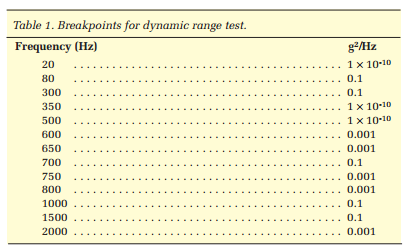For sake of comparison, the nonlinear method from Reference 3 was also computed for skewness = 0 and kurtosis = 5. Figure 6 shows that the spectrum with the new technique is unchanged by the increase in kurtosis, demonstrating that the same PSD with the same dynamic range is achieved regardless of kurtosis level.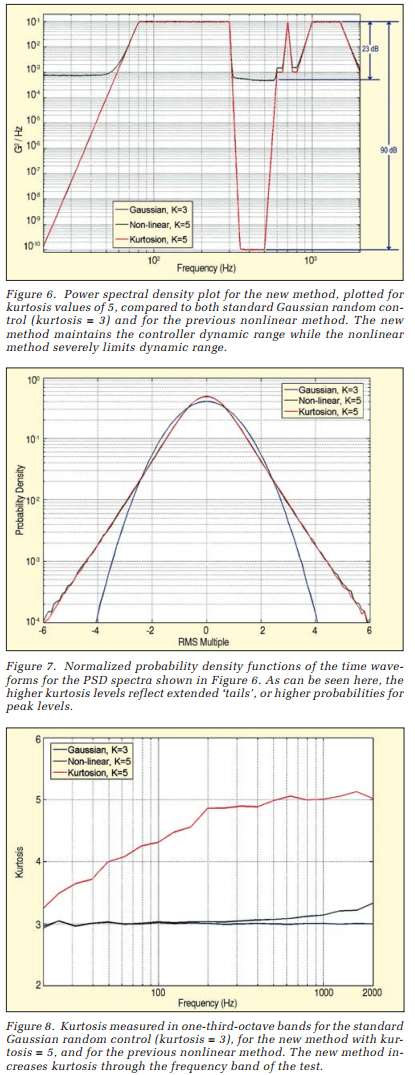In fact, the spectrum control is unchanged by the kurtosis change, so it is possible to change the kurtosis from 3 to 5 to 7 (and beyond) without any change in the resulting spectrum. In contrast, the previous nonlinear technique shows a significant loss in dynamic range, so that only 23 dB of dynamic range remains.

The normalized PDFs of the resulting time waveforms are shown in Figure 7. As can be seen here, higher kurtosis levels reflect extended ‘tails,’ or higher probabilities for the peak levels. When viewed from this perspective, the new technique and the previously proposed nonlinear technique yield similar results. To examine the relationship between kurtosis and frequency, the data were separated into one-third-octave bands, and the kurtosis of each band was computed. These results are plotted in Figure 8. We see that the new method increases the kurtosis across the entire frequency band for the test, while the previous method actually shows no increase over any single narrow band.

### Experimental Results

The obvious question is, “What significance does this have to vibration testing?” The bottom line in vibration testing is that the testing should highlight potential field failure problems early in development, before they become issues in the field. If including kurtosis does not make a difference in isolating product weaknesses, then it would be a meaningless statistic. With this in mind, we constructed a simple controlled experiment to investigate the effects of increased kurtosis on time to failure. Ordinary 75-watt light bulbs were chosen as the test item, because the failure of a light bulb is readily evident. Also, light bulbs are inexpensive, which allows for many test runs to improve the statistical significance of the results.

The PSD used was based on a standard automotive specification, referred to as “body and IP profile,” with a modification of extending the bandwidth of the low-frequency energy level out to 100 Hz to encompass the observed 90 Hz resonance frequency present in the light bulbs. The breakpoints for this profile are listed in Table 2.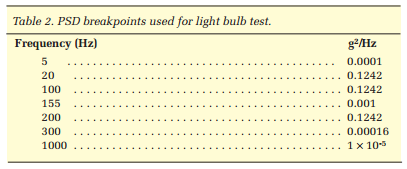A fixture with two light bulb sockets was mounted to the table of an electrodynamic shaker, as shown in Figure 9.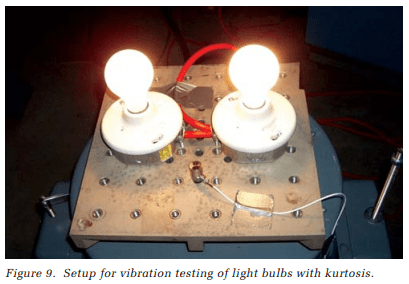Tests were performed on a total of 66 light bulbs, testing 22 bulbs each at kurtosis levels of 3, 5 and 7. While the vibration test was running, the current through the light bulbs was monitored and plotted on the display. As a light bulb begins to fail, the current would increase somewhat. When complete filament failure occurred, the current would drop suddenly. The time to failure was measured as the time from the beginning of the test to the time to the time of the sudden drop in current. Since two bulbs were tested simultaneously, the test was allowed to continue until both bulbs had failed, and then the time to failure was computed individually for each bulb. Sample screen shots from the VibrationVIEW software are shown in Figure 10. Note that the PSD was the same in all cases, while the higher kurtosis levels translated into higher peak levels and shorter time to failure.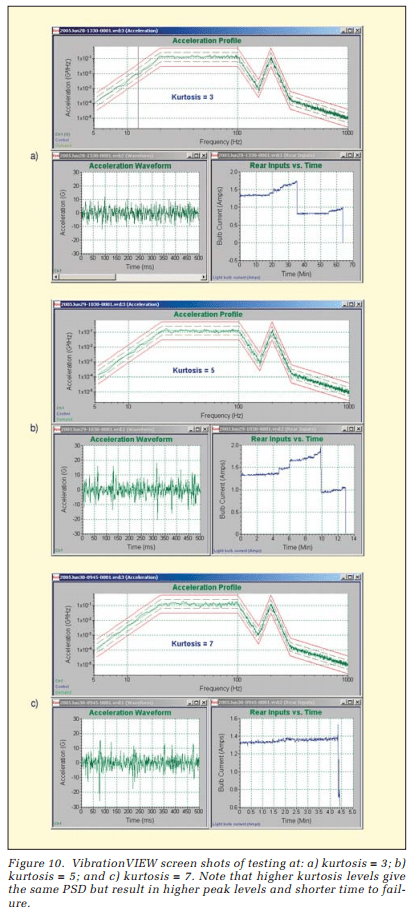The time to failure for all tests is shown in Figure 11. This figure shows that the time to failure decreased as kurtosis level increased. Taking the average time to failure of the 22 tests at each kurtosis level, it becomes readily evident that the light bulbs are brought to failure significantly faster when the kurtosis is increased, from 62 minutes with kurtosis = 3, to 16 minutes with kurtosis = 5, to 6.7 minutes with kurtosis = 7.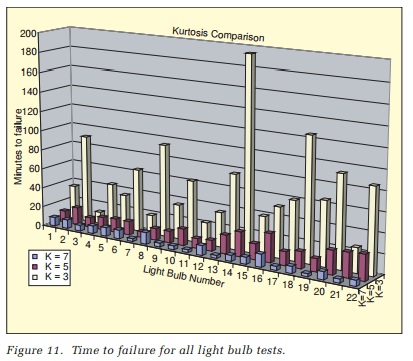The light bulb test performed here is a very simple example, but it clearly illustrates the point. Decreased time to failure means that failure modes are more readily produced in lab testing with higher kurtosis levels. It also means that you are more likely to detect potential field failures if you include the kurtosis parameter when performing vibration testing. Now that kurtosis can be easily included in random vibration tests, manufacturers can perform similar case studies on their components to determine how kurtosis can help them in designing more reliable parts.

### Conclusion

Kurtosis is an exciting new development for random vibration control. Technicians have wanted this realism factor for a long time, even if they did not know what kurtosis was. Before today, there has not been a way to add this important ‘peakyness’ factor to your random test. Vibration Research Corporation has demonstrated a method for reproducing not only the PSD and RMS levels, but also the kurtosis of the field data. We have developed, commercialized and applied for a patent on this technique. It is very easy to use. The kurtosis is easily measured from field data, and this measurement can simply be entered into the test specification. The method preserves all the important characteristics needed for random testing, such as spectrum shape, average RMS levels, and wide dynamic range, while at the same time controlling kurtosis level. Testing and design engineers are now free to include the desired kurtosis in their random vibration test specifications to increase the realism of their tests, improve the likelihood of detecting failure mechanisms during testing and therefore increase the reliability of their designs. Random vibration testing has never been better.

###### References

1. Connon, W. H., “Comments on Kurtosis of Military Vehicle Vibration Data,” Journal of the Institute of Environmental Sciences, September/October 1991, pp. 38-41.

2. Steinwolf, A., “Shaker Simulation of Random Vibration with a High Kurtosis Value,” Journal of the Institute of Environmental Sciences, May/June 1997, pp. 33-43.

3. Steinwolf, A. and Connon W. H., “Limitations on the use of Fourier Transform Approach to Describe Test Course Profiles,” Sound and Vibration, Feb. 2005, pp. 12-17.

###### Why Did the Light Bulbs Fail?

The widgets we used for our test were light bulbs. We brought the light bulbs to failure during the test. The next question an engineer will want to know is why did the light bulbs fail? To answer this question, we need to examine failure modes and other evidence. Inspect the bulb current graphs in Figure 10. Note that the current slowly rises from about 1.35 amps to 1.70 amps and then falls sharply as one of the two bulbs under test has failed. With only one bulb operating, the current drops to about 0.65 amp and slowly climbs to about 1.00 amp. Then the second bulb also fails, accompanied by a drop in current to 0.

A rising current must imply a decrease in resistance according to Ohm’s Law (E = IR). What accounts for the lowered resistance? An examination of the filaments of an unused 75-watt “Do-It” light bulb and of a tested 75-W “Do-It” light bulb (used in random testing with “body and IP” profile light bulb – four for 54 minutes) is enlightening.* The light bulb in Figure 1.2 started at 1.32 amps. At the time the light bulb failed during testing, the current had risen to 1.60 amps.

The tested light bulb filament shows many characteristics of deformation. At the top of the filament, the once tightly coiled filament has been stretched into a thin straight wire. At the bottom of the filament, the coils have become very closely bunched. So, what was the failure mechanism? Given more than one engineer, you will have more than one theory of failure. This is our current* situation.

Theory 1 – It seems that these deformities have had the multiplied effect of ‘shortening’ the filament and reducing the resistance. The shortening is caused by the filament looping on itself, causing the current to rise. Note the steps in the current before actual failure, caused by looping of the filament. Eventually, the increased current eventually finds a weak spot, causing a discontinuity of the filament.

Theory 2 – The originally coiled filament heats evenly, causing a stable resistance. As the filament stretches out, it begins to cool as the energy is radiated away from the adjacent filament coils. A cooler filament has lower resistance. A lower resistance will increase the current, eventually causing a hot spot and a failure. The steps in current before failure are likely caused by high g peaks stretching the coil out suddenly.

If you would like to vote on these theories, have another theory of the failure mechanism, or wish to suggest a test to end all theories, please mail it to us, written on an unused 75- watt light bulb. We need more bulbs for testing.

* Pun intended

Appeared in: Sound & Vibration Magazine, October 2005 pp 2-7.